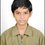# What is wrong in this?

1 = (1 x 3)/3
1 = (0.333333333....33) x 3
1 = 0.99999..999
=> 0.0000....001 = 0
=> 1/10^ ∞ = 0
=> 1 = 0Note by Ksg Sarma
5 years, 1 month ago

This discussion board is a place to discuss our Daily Challenges and the math and science related to those challenges. Explanations are more than just a solution — they should explain the steps and thinking strategies that you used to obtain the solution. Comments should further the discussion of math and science.

When posting on Brilliant:

• Use the emojis to react to an explanation, whether you're congratulating a job well done , or just really confused .
• Ask specific questions about the challenge or the steps in somebody's explanation. Well-posed questions can add a lot to the discussion, but posting "I don't understand!" doesn't help anyone.
• Try to contribute something new to the discussion, whether it is an extension, generalization or other idea related to the challenge.

MarkdownAppears as
*italics* or _italics_ italics
**bold** or __bold__ bold
- bulleted- list
• bulleted
• list
1. numbered2. list
1. numbered
2. list
Note: you must add a full line of space before and after lists for them to show up correctly
paragraph 1paragraph 2

paragraph 1

paragraph 2

[example link](https://brilliant.org)example link
> This is a quote
This is a quote
    # I indented these lines
# 4 spaces, and now they show
# up as a code block.

print "hello world"
# I indented these lines
# 4 spaces, and now they show
# up as a code block.

print "hello world"
MathAppears as
Remember to wrap math in $$ ... $$ or $ ... $ to ensure proper formatting.
2 \times 3 $2 \times 3$
2^{34} $2^{34}$
a_{i-1} $a_{i-1}$
\frac{2}{3} $\frac{2}{3}$
\sqrt{2} $\sqrt{2}$
\sum_{i=1}^3 $\sum_{i=1}^3$
\sin \theta $\sin \theta$
\boxed{123} $\boxed{123}$

Sort by:

First off, $0 \times \infty$ isn't defined. For more info, refer to indeterminate forms.

Secondly, the last equation should be $\lim_{n \to \infty} \frac{1}{10^n} = 0$

Rather than $\frac{1}{10^{\infty}} = 0$

Also, note that $\lim_{n \to \infty} 0 \times 10^n = 0$

But, $\lim_{n \to \infty} \frac{1}{10^n} = 0 \not \Rightarrow \lim_{n \to \infty} 0 \times 10^n = 1$

- 5 years, 1 month ago

1/10^ ∞ is not exactly equal to 0 its value is too small and greater than 0 so when i multiply it with 0 it should be 0 . And according to your 1st statement 1 is undefined

- 5 years, 1 month ago

$\dfrac{1}{10^{\infty}}$ isn't even a number!

- 5 years, 1 month ago

moreover the main problem is with 3rd step where i am getting 1 is exactly equal to 0.999999..9999

- 5 years, 1 month ago

There are so many errors that you've made in the above note.

First error

The $.33333....333$ you wrote implies that the $3$s will end since the last 3 isn't followed by $...$ to imply that it repeats infinitely

Second error

This follows from your first and is very similar.Again.you're implying that the $9$s will end sometime,which they don't because the 9s repeat infinitely.(occurs in line 3)

Third error

This doesn't even make sense.If something equals something,subtracting them from each other will result in exactly $0$.This follows from your other errors too.(occurs in line 4)

Fourth error

You can't put $.000..1$ equal to $\frac{1}{10^{\infty}}$ because you can't do arithmetic with infinity,it's a concept not a number.

Fifth error

Let's assume you used the correct way to express it which is $\lim _{n \rightarrow \infty}{\frac{1}{10^{n}}}=0$,you can't multiply each side by $10^n$ since it's in the limit,and it's not a constant.

- 5 years, 1 month ago

in the 1st error you said , tell me how many no. of 3's are there in the middle then I agree that it will end and all the errors are similar

- 5 years, 1 month ago

the 3s don't end,they repeat infinitely

- 5 years, 1 month ago

$0.999\ldots = 1$ exactly!

- 5 years, 1 month ago

You can't play with infinity digit number like that!

- 5 years, 1 month ago

why don't u just make wiki

- 5 years, 1 month ago